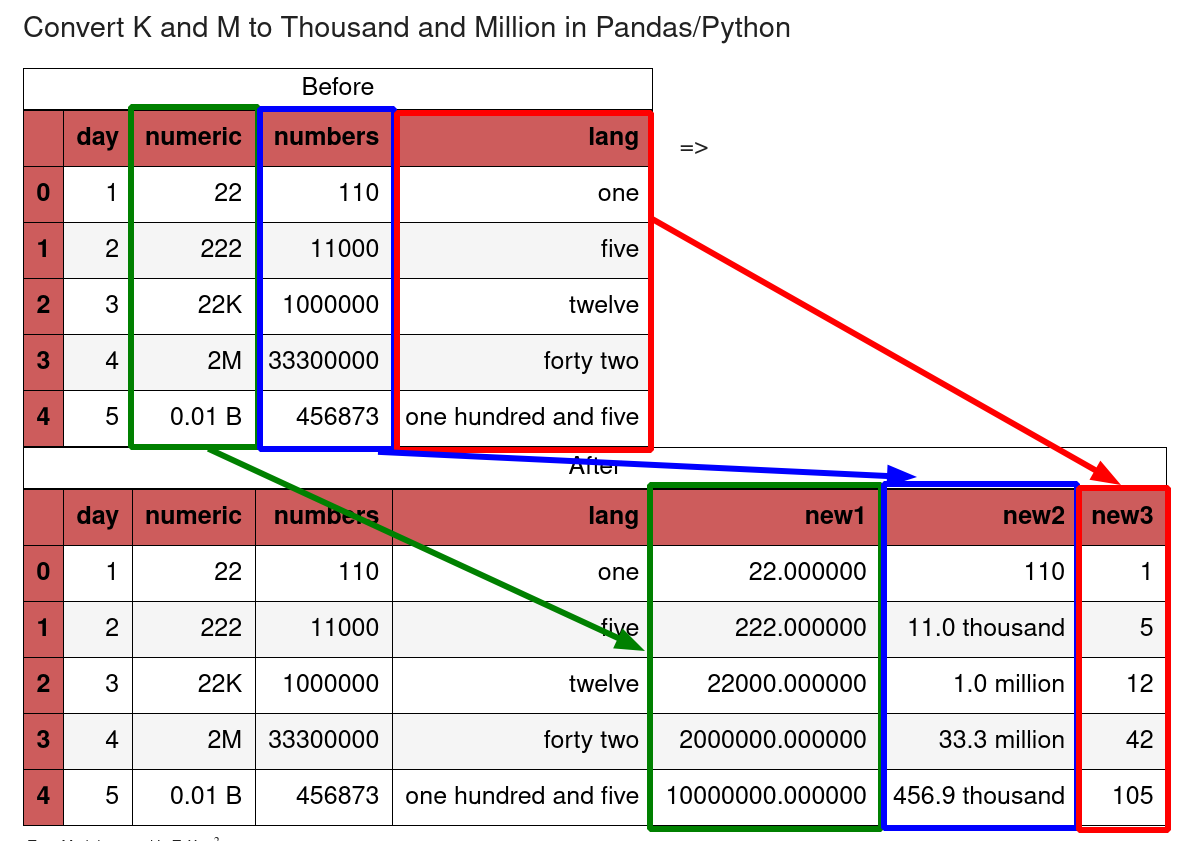In this short tutorial, we'll cover how to convert natural language numerics like M and K into numbers with Pandas and Python.

We will show two different ways for conversion of K and M to thousand and million. We will also cover the reverse case converting thousand and million to K and M.

So we will cover:

• 0.1M to 100000
• 1 K - 1000
• 10000 to 10K
• forty two - 42

Two Python libraries:

• humanize - turning a number into a fuzzy human-readable expression
• numerizer - convert natural language numerics into ints and floats

The image below show the examples:## Setup

Let's use the following DataFrame the conversion from natural language numerals to numbers:

``````import pandas as pd
import matplotlib.pyplot as plt

data={'day': [1, 2, 3, 4, 5],
'numeric': [22, 222, '22K', '2M', '0.01 B'],
'numbers': [110, 11000, 1000000, 33300000, 456873],
'lang': ['one', 'five', 'twelve', 'forty two', 'one hundred and five']}

df = pd.DataFrame(data,
columns=['day', 'numeric', 'numbers', 'lang'])
``````

Our data looks like:

day numeric numbers lang
0 1 22 110 one
1 2 222 11000 five
2 3 22K 1000000 twelve
3 4 2M 33300000 forty two
4 5 0.01 B 456873 one hundred and five

## Step 1: Convert K/M to Thousand/Million

First we will start the conversion of large number abbreviations to numbers. We will map the abbreviations to the math expression.

So we will convert:

``````22K -> 22 * 10**3
0.1 M -> 0.1 * 10**6
``````
``````mp = {'K':' * 10**3', 'M':' * 10**6', 'B':' * 10**9', 't':' * 10**12', 'q':' * 10**15', 'Q':' * 10**15'}
pd.eval(df['numeric'].replace(mp.keys(), mp.values(), regex=True))
``````

This will give us:

``````array([22.0, 222.0, 22000.0, 2000000.0, 10000000.0], dtype=object)
``````

As we can see it works fine for columns with mixed data. It works well also for numeric values with spaces like `1 M`

## Step 2: Convert Thousand/Million to K/M

For this step we will use the Python library - `humanize`. It will help us to** convert easily and reliably large numbers to human readable abbreviations**:

``````import humanize
df['numbers'].apply(humanize.intword)
``````

The result contains the converted values:

``````0               110
1     11.0 thousand
2       1.0 million
3      33.3 million
4    456.9 thousand
Name: numbers, dtype: object
``````

The library supports multiple languages - about 25 like:

• spanish
• russian
• french
• portuguese

Library can be installed by:

``````pip install humanize
``````

## Step 3: Convert words to number - one to 1

Finally let's cover the case when we need to convert language numerics into numbers:

• forty two -> 42
• twelve hundred -> 12000
• four hundred and sixty two -> 462

This time we will use Python library: numerize - which can be installed by:

``````pip install numerize
``````

So the Pandas code to convert numbers is:

``````from numerizer import numerize
df['lang'].apply(numerize)
``````

The output is:

``````0      1
1      5
2     12
3     42
4    105
Name: lang, dtype: object
``````

## Large Number Abbreviations

Below we can find a table of large number abbreviations which can be used to improve the mapping.

Abbreviation Name Value Equivalent
K Thousand (Kilo) 10^ 3 1000
M Million 10^ 6 1000K
B Billion 10^ 9 1000M
t trillion 10^ 12 1000B